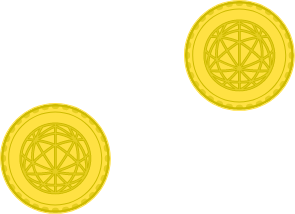# 38 of 100: Curse of the Forgetful Cheater

Probability Level 2You have two coins that look identical, but one of them is fair and the other is weighted. The weighted coin has a $\frac{3}{4}$ chance of flipping heads and a $\frac{1}{4}$ chance of flipping tails.

Unfortunately, you've forgotten which coin is which! You decide to keep flipping them together, one in each hand, until you get a flip where one coin shows heads and the other shows tails. Then you'll assume that the coin showing heads is the weighted coin. If you do this, what's the probability you'll correctly identify the coins?Good luck, this is a very tricky question! Remember that a fair coin is one that has a $\frac{1}{2}$ chance of flipping heads and a $\frac{1}{2}$ chance of flipping tails.

×